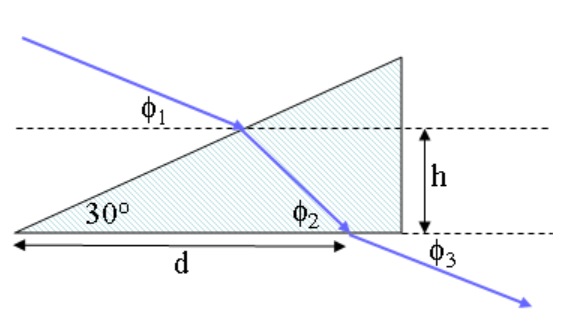# Problem: Red light is incident in air on a 30- 60- 90(degree) prism as shown. The incident beam is directed at an angle of ϕ1 = 41.3° and ϕ2 = 46.6° with respect to the horizontal and enters the prism at a height h = 17 cm above the base. The beam leaves the prism to the air at a distance d = 45.5 along the base as shown. The refractive index of the glass is 1.39.What is ϕ3, the angle the transmitted beam makes with the horizontal axis?

###### FREE Expert Solution

Snell's law:

$\overline{){{\mathbf{\eta }}}_{{\mathbf{1}}}{\mathbf{s}}{\mathbf{i}}{\mathbf{n}}{{\mathbf{\theta }}}_{{\mathbf{1}}}{\mathbf{=}}{{\mathbf{\eta }}}_{{\mathbf{2}}}{\mathbf{s}}{\mathbf{i}}{\mathbf{n}}{{\mathbf{\theta }}}_{{\mathbf{2}}}}$

η1 = ηglass = 1.39

η2 = ηair = 1

99% (477 ratings)###### Problem Details

Red light is incident in air on a 30- 60- 90(degree) prism as shown. The incident beam is directed at an angle of ϕ1 = 41.3° and ϕ2 = 46.6° with respect to the horizontal and enters the prism at a height h = 17 cm above the base. The beam leaves the prism to the air at a distance d = 45.5 along the base as shown. The refractive index of the glass is 1.39.What is ϕ3, the angle the transmitted beam makes with the horizontal axis?

Frequently Asked Questions

What scientific concept do you need to know in order to solve this problem?

Our tutors have indicated that to solve this problem you will need to apply the Refraction Of Light concept. You can view video lessons to learn Refraction Of Light. Or if you need more Refraction Of Light practice, you can also practice Refraction Of Light practice problems.